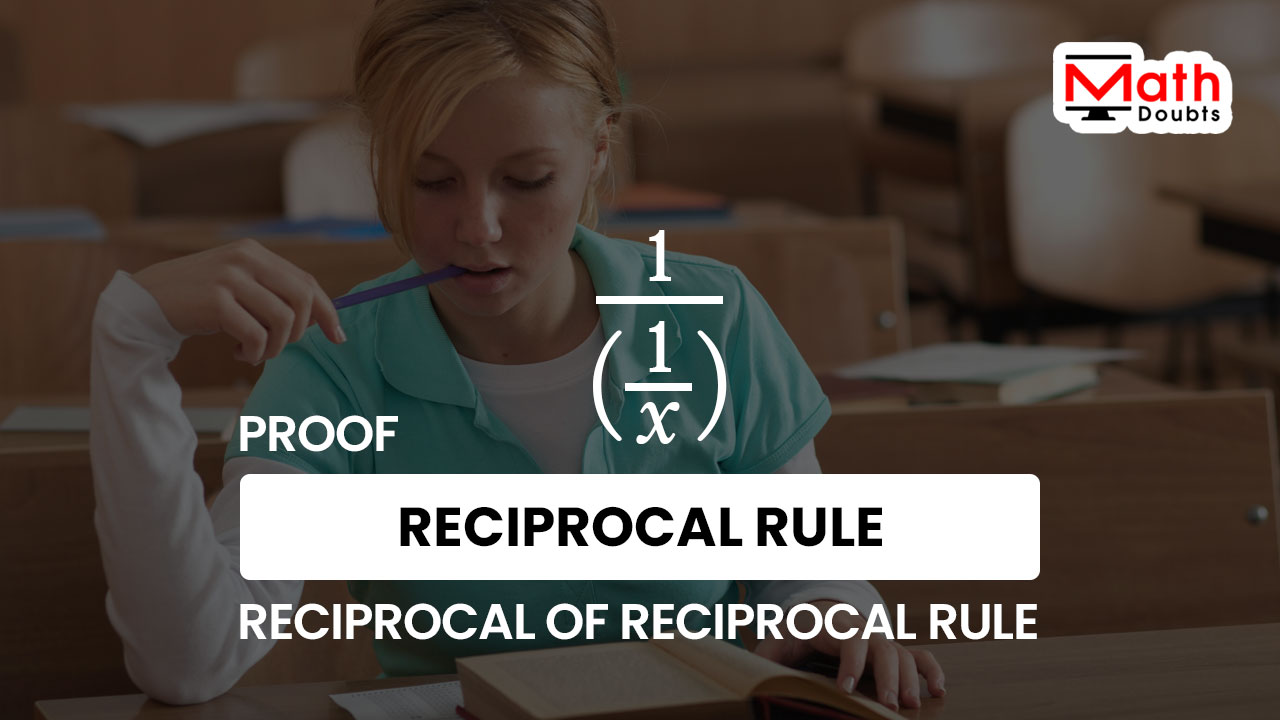# Proof of Reciprocal of a Reciprocal rule

The reciprocal of a reciprocal rule expresses that the reciprocal of the multiplicative inverse of a variable $x$ is exactly equal to the value of the variable $x$.

$\dfrac{1}{\bigg(\dfrac{1}{x}\bigg)}$ $\,=\,$ $x$Let’s learn how to derive the reciprocal rule of a reciprocal function mathematically in algebraic form.

### Denote the Reciprocal of fraction by a variable

Let’s denote the multiplicative inverse of the reciprocal of a variable $x$ by a variable $y$.

$\dfrac{1}{\bigg(\dfrac{1}{x}\bigg)}$ $\,=\,$ $y$

### Simplify Rational equation by Cross multiplication

The expression on the left hand side of the equation is a rational function. So, let’s write the expression on the right hand side of the equation as a rational function.

$\implies$ $\dfrac{1}{\bigg(\dfrac{1}{x}\bigg)}$ $\,=\,$ $\dfrac{y}{1}$

Now, the expressions on both sides of the equation are in rational form. So, the equation can be simplified by the cross multiply property.

$\implies$ $1 \times 1$ $\,=\,$ $y \times \bigg(\dfrac{1}{x}\bigg)$

It is time to multiply the factors on both sides of the equation. Firstly, let’s concentrate on the arithmetic expression on the left hand side of the equation. The one time one is equal to one.

$\implies$ $1$ $\,=\,$ $y \times \dfrac{1}{x}$

Now, focus on the expression on the right hand side of the equation. The variable $y$ is not a fraction but the second factor is a fraction. So, it is better to express the first factor as a fraction.

$\implies$ $1$ $\,=\,$ $\dfrac{y}{1} \times \dfrac{1}{x}$

The two factors are now fractions and their product can be evaluated by the multiplication of the fractions.

$\implies$ $1$ $\,=\,$ $\dfrac{y \times 1}{1 \times x}$

$\implies$ $1$ $\,=\,$ $\dfrac{y}{x}$

There is a fraction on the right hand side but there is an integer on the left hand side of the equation. So, expressing the integer $1$ as a rational number helps us to use the cross multiplication rule in simplifying the equation.

$\implies$ $\dfrac{1}{1}$ $\,=\,$ $\dfrac{y}{x}$

Now, the equation in rational form can be simplified by using the cross multiply formula.

$\implies$ $1$ $\times$ $x$ $\,=\,$ $y \times 1$

$\,\,\,\therefore\,\,\,\,\,\,$ $x$ $\,=\,$ $y$

It is proved that the value of variable $x$ is exactly equal to the value of the variable $y$.

### Obtain the formula by replacing the variable

The variable $y$ is considered to represent the reciprocal of the reciprocal of $x$. So, the variable $y$ can be eliminated from the equation by replacing the variable $y$ with its value in $x$.

$\implies$ $x$ $\,=\,$ $\dfrac{1}{\bigg(\dfrac{1}{x}\bigg)}$

$\,\,\,\therefore\,\,\,\,\,\,$ $\dfrac{1}{\bigg(\dfrac{1}{x}\bigg)}$ $\,=\,$ $x$

Therefore, it is proved that the reciprocal of the reciprocal of a quantity is exactly equal to the value of the quantity.

A best free mathematics education website that helps students, teachers and researchers.

###### Maths Topics

Learn each topic of the mathematics easily with understandable proofs and visual animation graphics.

###### Maths Problems

A math help place with list of solved problems with answers and worksheets on every concept for your practice.

Learn solutions

###### Subscribe us

You can get the latest updates from us by following to our official page of Math Doubts in one of your favourite social media sites.